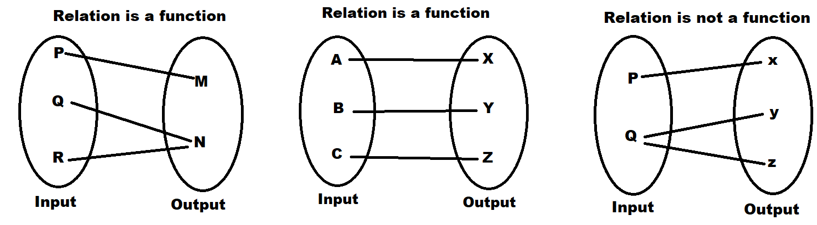Maths-
General
Easy

Question

# How can you determine whether a relation is a function ?Hint:

## The correct answer is: independent variable.

### Consider the following set of ordered pairs. The first numbers in each pair are the first five natural numbers. The second number in each pair is twice that of the first.{(1, 2),(2, 4),(3, 6),(4, 8),(5, 10)}The domain is {1,2,3,4,5}. The range is {2,4,6,8,10}.Note that each value in the domain is also known as an input value, or independent variable. Each value in the range is also known as an output value, or dependent variable.A function, f, is a relation that assigns a single value in the range to each value in the domain. In other words, no x-values are repeated.For our example that relates the first five natural numbers to numbers double their values, this relation is a function because each element in the domain, {1,2,3,4,5}, is paired with exactly one element in the range, {2,4,6,8,10}.Now let’s consider the set of ordered pairs that relates the terms “even” and “odd” to the first five natural numbers. It would appear as{(odd, 1),(even, 2),(odd, 3),(even, 4),(odd, 5)}Notice that each element in the domain, {even, odd} is not paired with exactly one element in the range, {1,2,3,4,5}. For example, the term “odd” corresponds to three values from the domain, {1,3,5} and the term “even” corresponds to two values from the range, {2,4}. This violates the definition of a function, so this relation is not a function.Each value in the domain is also known as an input value, or independent variable. Each value in the range is also known as an output value, or dependent variable.#### With Turito Foundation.#### Get an Expert Advice From Turito.